For those wishing to follow along with the R-based demo in class, click here for the companion R script for this lecture.

In this course so far, we have constructed data-generating models and fitted these models to observed data using likelihood-based methods (ML and Bayesian inference). We also have explored a range of methods to account for structural uncertainty (which of a set of candidate models could plausibly have generated our observed data).

But even after we have fitted a model to data, even after we have compared a suite of plausible models and selected the best one, are we really sure that the model is good?

What does it even mean to say that the model is good?

Usually, we mean one or more of the following:

1. Goodness-of-fit: The data could easily/reasonably have been generated under the fitted model (the model is adequate)
2. Predictive performance: The fitted model performs well at predicting responses for out-of-sample data (the model is useful)
3. Generality: The model performs well at extrapolating responses for out-of-sample data that are outside the range of those in the training set (the model is really useful!)

## Goodness-of-fit

We have already looked at a variety of methods for evaluating goodness-of-fit. In general, you can use data simulation (virtual ecology) to evaluate whether your fitted model is capable of generating the observed data. In a frequentist/ML context this is often called a parametric bootstrap

Q: What is the difference between a non-parametric bootstrap (usually just called a ‘bootstrap’) and a parametric bootstrap?

For example, we can overlay the observed data (or a model perforance statistic like R-squared) on a cloud of points representing the range of data sets (or performance statistics) possibly produced under the fitted model.

Or, we can use a “plug-in” prediction bounds as a substitute for the cloud of data sets possibly produced under the model.

Often, we compare a target summary statistic (e.g., Deviance, RMSE, R-squared) for the observed data with the range of that target summary statistic produced under the inferred, stochastic data generating model.

library(emdbook)

MyxDat <- MyxoTiter_sum
head(Myx)
##   grade day titer
## 1     1   2 5.207
## 2     1   2 5.734
## 3     1   2 6.613
## 4     1   3 5.997
## 5     1   3 6.612
## 6     1   3 6.810

Now let’s use ML to fit the Ricker model with a gamma error distribution.

#######
# Fit the model with ML
#######

Ricker <- function(a,b,predvar) a*predvar*exp(-b*predvar)

NegLogLik_func <- function(params,data){
expected <- Ricker(params,params,data$day) -sum(dgamma(data$titer,shape=params,scale=expected/params,log = T))
}

init.params <- c(a=1,b=0.2,shape=50)
NegLogLik_func(init.params,data=Myx)
##  2336.22
MaxLik <- optim(par=init.params, fn=NegLogLik_func, data=Myx)

MaxLik
## $par ## a b shape ## 3.5614933 0.1713346 90.6790545 ## ##$value
##  29.50917
##
## $counts ## function gradient ## 202 NA ## ##$convergence
##  0
##
## $message ## NULL How might we evaluate goodness-of-fit in this case?? ### ‘Plug-in’ prediction bounds The simplest way is just to plot the expected value along with “plug-in” bounds around that prediction, to represent the range of data likely to be produced under the model. We have done this before! ######## # Plug-in prediction interval ######## plot(Myx$titer~Myx$day,xlim=c(0,10),ylim=c(0,15)) expected <- Ricker(MaxLik$par['a'],MaxLik$par['b'],1:10) points(1:10,expected,type="l",col="green") upper <- qgamma(0.975,shape=MaxLik$par['shape'],scale=expected/MaxLik$par['shape']) lower <- qgamma(0.025,shape=MaxLik$par['shape'],scale=expected/MaxLik$par['shape']) points(1:10,upper,type="l",col="red",lty=2) points(1:10,lower,type="l",col="red",lty=2)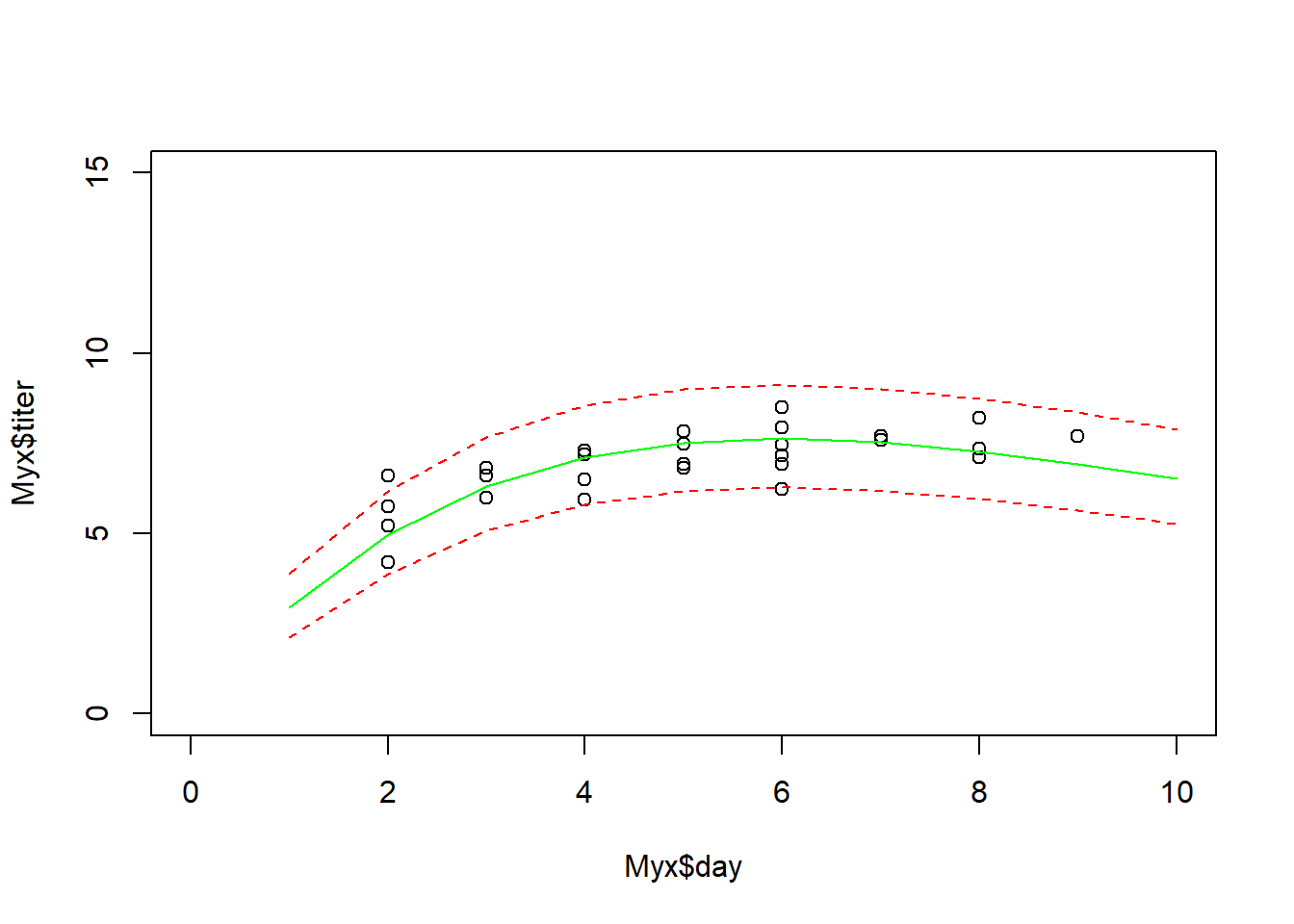This gives us a simple and useful way to visualize goodness-of-fit. ### Simulated datasets! Alternatively, we could generate simulated data sets under the best-fit model (parametric bootstrap!): ######### # Parametric bootstrap! ######### plot(Myx$titer~Myx$day,xlim=c(0,10),ylim=c(0,15),type="n") expected <- Ricker(MaxLik$par['a'],MaxLik$par['b'],1:10) points(1:10,expected,type="l",col="green") uniquedays <- sort(unique(Myx$day))
expected <- Ricker(MaxLik$par['a'],MaxLik$par['b'],uniquedays)
simdata <- array(0,dim=c(1000,length(uniquedays)))
for(i in 1:1000){
simdata[i,] <- rgamma(length(uniquedays),shape=MaxLik$par['shape'],scale=expected/MaxLik$par['shape'])
}

upper <- apply(simdata,2,function(t) quantile(t,0.975))
lower <- apply(simdata,2,function(t) quantile(t,0.025))

points(uniquedays,upper,type="l",col="red",lty=2)
points(uniquedays,lower,type="l",col="red",lty=2)

points(Myx$day,Myx$titer,cex=1.5,pch=20)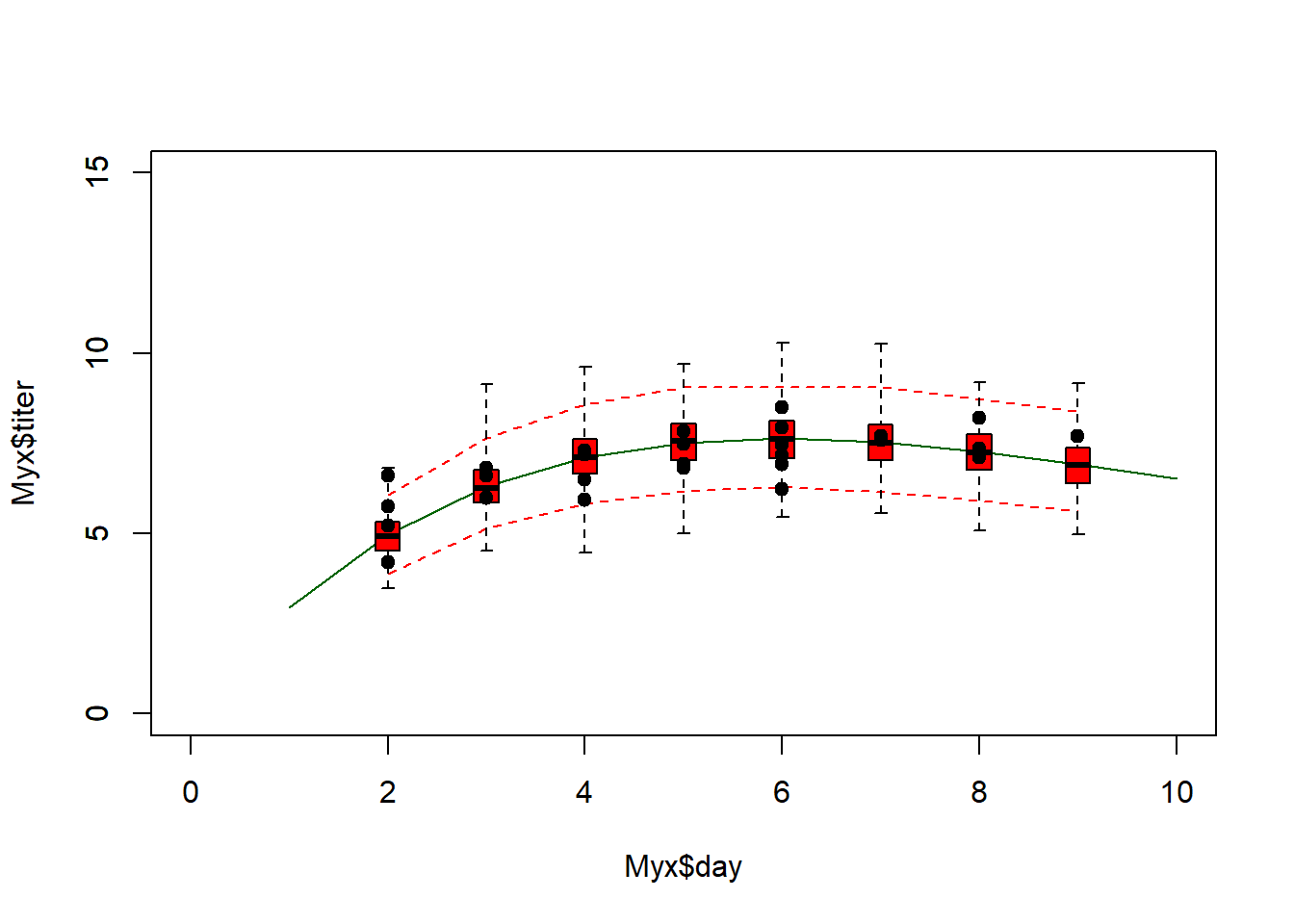Using simulated data, we can go further- we can compare the simulated data vs the observed data more quantitatively. For example, we can compute the root mean squared error (RMSE) for the simulated datasets and compare that with the root mean squared error for the observed data.

########
# Compare observed error statistic with expected range of error statistic as part of parametric bootstrap analysis

expected <- Ricker(MaxLik$par['a'],MaxLik$par['b'],Myx$day) simdata <- array(0,dim=c(1000,length(Myx$day)))
for(i in 1:1000){
simdata[i,] <- rgamma(length(Myx$day),shape=MaxLik$par['shape'],scale=expected/MaxLik$par['shape']) } rmse_observed <- sqrt(mean((Myx$titer-expected)^2))
rmse_simulated <- apply(simdata,1,function(t) mean((t-expected)^2))

hist(rmse_simulated,freq=F)
abline(v=rmse_observed,col="green",lwd=3)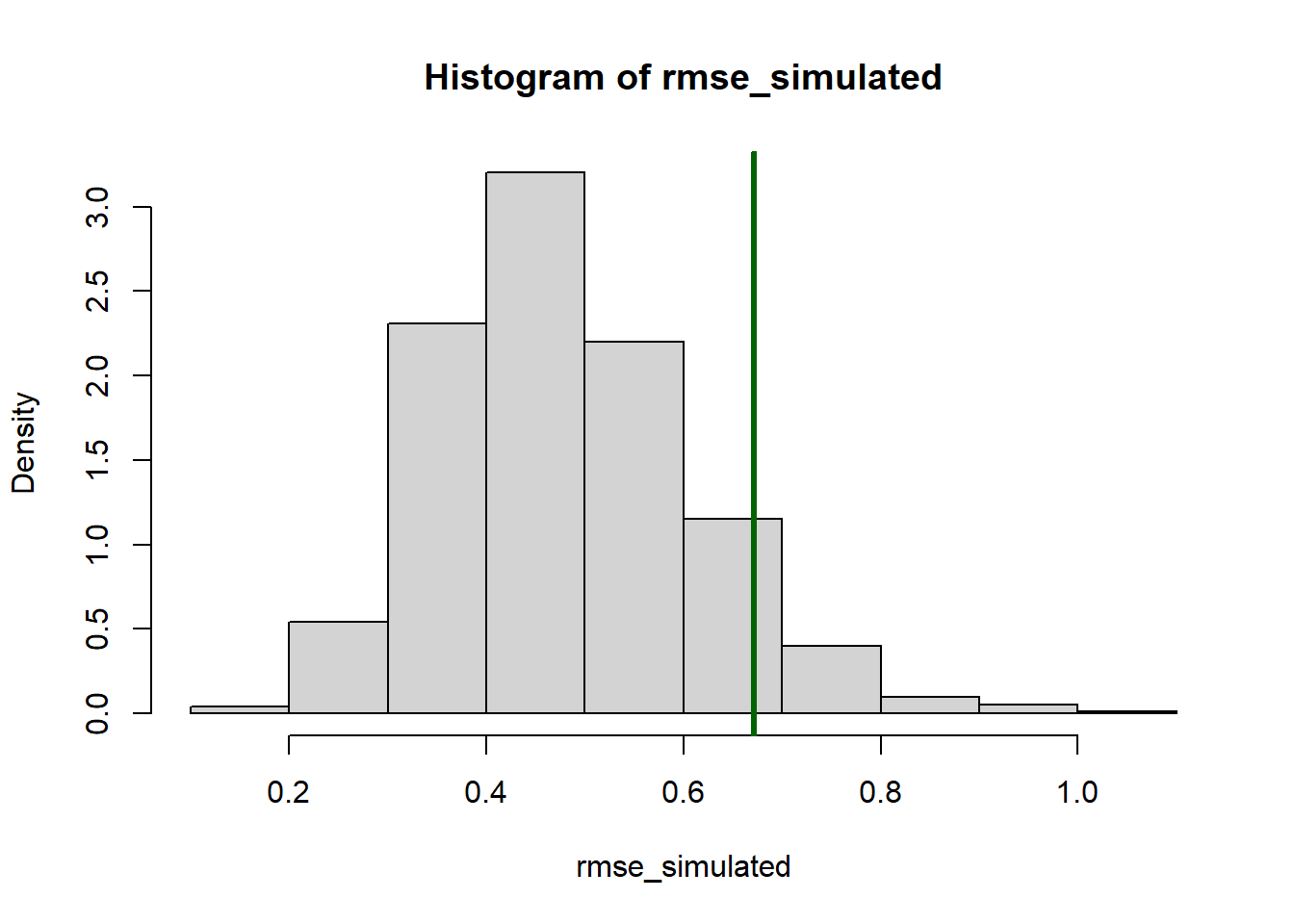So, is this a good model?

Of course, the goodness-of-fit tests you can run with simulated data are limited only by your imagination! Be dangerous!

NOTE: the above methods do not account for parameter uncertainty. This model is the maximum likelihood model- that is, the point estimates are assumed to represent the true model!

If we wanted to account for parameter uncertainty in the ML framework, we could (for example) use profile-likelihood CIs to estimate parameter bounds and we could plug-in bounds that incorporate parameter uncertainty. Or we could treat the CIs for the parameter estimates as a probability distribution (e.g., uniform) and simulate datasets across this range of parameter uncertainty (but what would a frequentist statistician say about this?).

### Bayesian!

Accounting for parameter uncertainty in simulations is simple in a Bayesian framework… (this is part of lab 4!)

In general, a posterior predictive check involves generating new data sets under the fitted model that are equivalent to the observed data set (i.e., same sample size and covariate values) and comparing with the observed data.

First we need to fit the model in JAGS.

Note that we can write the predictions directly into the JAGS code

#########
# Bayesian goodness-of-fit
#########

library(R2jags)
## Loading required package: rjags
## Loading required package: coda
## Linked to JAGS 4.2.0
## Loaded modules: basemod,bugs
##
## Attaching package: 'R2jags'
## The following object is masked from 'package:coda':
##
##     traceplot
library(lattice)

cat("
model {

#############
# LIKELIHOOD
############
for(obs in 1:n.observations){
expected[obs] <- a*day[obs]*exp(-b*day[obs])  # Ricker
titer[obs] ~ dgamma(shape,shape/expected[obs])
titer.sim[obs] ~ dgamma(shape,shape/expected[obs])    # simulate new data (accounting for parameter uncertainty!
}

#############
# PRIORS
############
shape ~ dgamma(0.001,0.001)
a ~ dunif(0,10)
b ~ dunif(0,10)

#############
# SIMULATED DATA FOR VISUALIZATION
#############

for(day2 in 1:10){
expected.new[day2] <- a*day2*exp(-b*day2)  # Ricker
titer.new[day2] ~ dgamma(shape,shape/expected.new[day2])
}

#############
# DERIVED QUANTITIES
#############
for(obs in 1:n.observations){
SE_obs[obs] <- pow(titer[obs]-expected[obs],2)
SE_sim[obs] <- pow(titer.sim[obs]-expected[obs],2)
}

RMSE_obs <- sqrt(mean(SE_obs[]))
RMSE_sim <- sqrt(mean(SE_sim[]))
}
", file="BUGSmod_ricker1.txt")

Let’s run the model!

myx.data.for.bugs <- list(
titer = Myx$titer, day = Myx$day,
n.observations = length(Myx$titer) ) init.vals.for.bugs <- function(){ list( shape=runif(1,20,100), a=runif(1,0.5,1.5), b=runif(1,0.1,0.3) ) } params.to.store <- c("shape","a","b","RMSE_obs","RMSE_sim","titer.new") # specify the parameters we want to get the posteriors for jags.fit <- jags(data=myx.data.for.bugs,inits=init.vals.for.bugs,parameters.to.save=params.to.store,n.iter=50000,model.file="BUGSmod_ricker1.txt",n.chains = 3,n.burnin = 5000,n.thin = 20 ) ## module glm loaded ## Compiling model graph ## Resolving undeclared variables ## Allocating nodes ## Graph information: ## Observed stochastic nodes: 27 ## Unobserved stochastic nodes: 40 ## Total graph size: 458 ## ## Initializing model jags.fit.mcmc <- as.mcmc(jags.fit) posterior <- as.data.frame(jags.fit$BUGSoutput$sims.list) Assuming convergence, let’s move on to the goodness-of-fit part! First, let’s visualize the observed data against the cloud of data that could be produced under this model! plot(Myx$titer~Myx$day,xlim=c(0,10),ylim=c(0,15),type="n") expected <- Ricker(mean(posterior$a),mean(posterior$b),1:10) points(1:10,expected,type="l",col="red") boxplot(x=as.list(posterior[,7:16]),at=1:10,add=T,boxwex=0.25,xaxt="n",range=0,border="red") points(Myx$day,Myx$titer,cex=1.5,pch=20)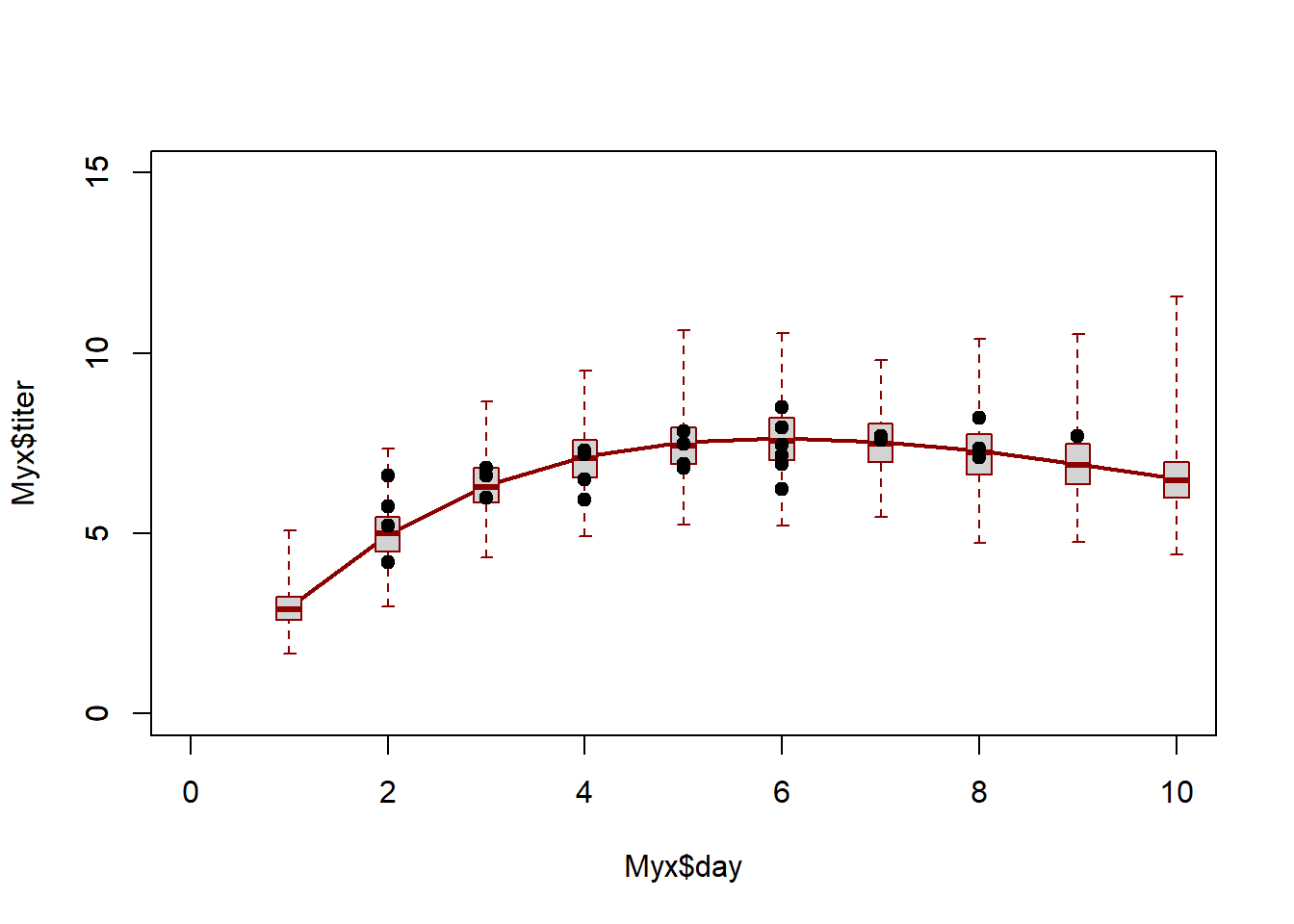Looks pretty good so far! Let’s look at another posterior predictive check… plot(posterior$RMSE_sim~posterior$RMSE_obs, main="posterior predictive check") abline(0,1,col="red",lwd=2)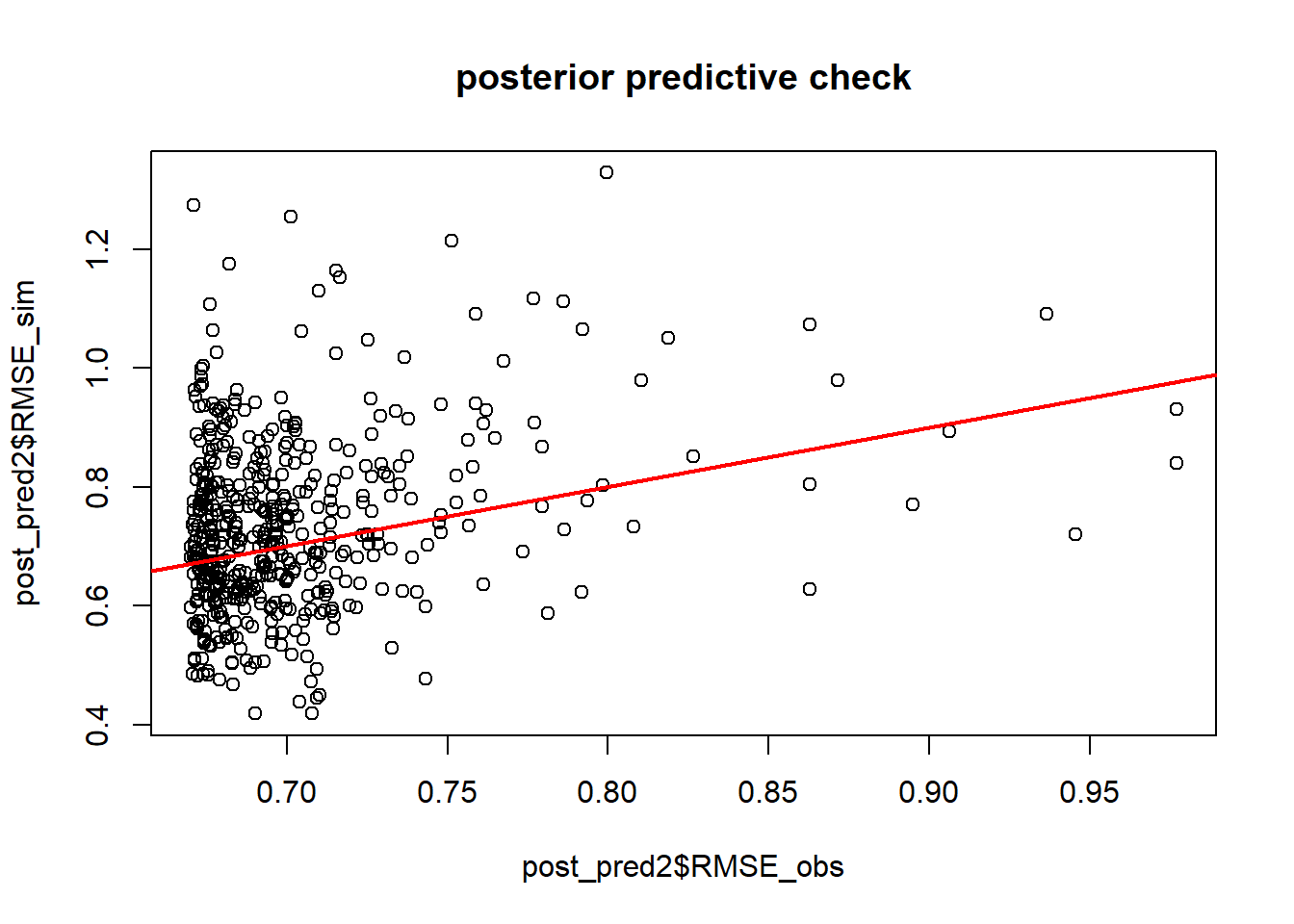p.value=length(which(as.vector(jags.fit.mcmc[,"RMSE_sim"][])>as.vector(jags.fit.mcmc[,"RMSE_obs"][])))/length(as.vector(jags.fit.mcmc[,"RMSE_sim"][])) p.value ##  0.6595556 Okay, the fit seems more or less reasonable! ## Predictive ability In many cases, goodness-of-fit is not ultimately what we are most interested in. What we really want to know is whether the model does a good job at predicting the response. Is our model useful in some way? One way to do this would be for us to focus on an R-squared or pseudo-R2 statistic: $$R^2 = 1-\frac{SS_{res}}{SS_{tot}}$$ $$PseudoR^2 = 1-(\frac{logLik_{mod}}{logLik_{null}})$$ Let’s compute these metrics for the fitted data: ########## # Summary statistics of a models "usefulness" (e.g., R-squared) SS_res <- sum((Myx$titer-Ricker(MaxLik$par["a"],MaxLik$par["b"],Myx$day))^2) SS_tot <- sum((Myx$titer-mean(Myx$titer))^2) Rsquared <- 1-SS_res/SS_tot cat("R-squared = ", Rsquared, "\n") ## R-squared = 0.46578 ######### # Fit the null likelihood model! NegLogLik_null <- function(params){ -sum(dgamma(Myx$titer,shape=params,scale=params/params,log = T))
}

init.params <- c(mean=7,shape=50)

MaxLik_null <- optim(par=init.params, fn=NegLogLik_null)

McFadden <- 1-(MaxLik$value/MaxLik_null$value)
cat("McFadden's R-squared = ", McFadden) 
## McFadden's R-squared =  0.2165806

Another way to evaluate model skill, or performance, is to use root mean squared error (RMSE):

$$RMSE = sqrt(mean(residuals^2))$$

RMSE gives a good indicator of the mean error rate, which is often useful and interpretable in an absolute way. For example, “the predicted temperature is usually within 1.3 degrees C of the true temperature”…

RMSE = sqrt(mean((Myx$titer-Ricker(MaxLik$par["a"],MaxLik$par["b"],Myx$day))^2))
cat("RMSE = ", RMSE, "\n")
## RMSE =  0.6767347

So the average error in units of titer (in log10 rabbit infectious doses)

So is our model good???

Does it do a good job at prediction?

Q: Does an over-fitted model have acceptable goodness-of-fit?

Q: Does an over-fitted model have acceptable performance, evaluated as R-squared or RMSE?

Q: Will an over-fitted model perform well when predicting to new samples (that were not used in model fitting)?

### Validation

Let’s imagine we collect some new Myxomatosis titer data, and it looks like this:

####
# Collect new data that were not used in model fitting

newdata <- data.frame(
day = c(2,3,4,5,6,7,8),
titer = c(4.4,7.2,6.8,5.9,9.1,8.3,8.8)
)
newdata
##   grade day titer
## 1     1   2   4.4
## 2     1   3   7.2
## 3     1   4   6.8
## 4     1   5   5.9
## 5     1   6   9.1
## 6     1   7   8.3
## 7     1   8   8.8

First we might simply visualize the new data against the cloud of data possibly produced under the fitted model…

###########
# Validation #1

plot(Myx$titer~Myx$day,xlim=c(0,10),ylim=c(0,15),type="n",xlab="days",ylab="titer")
expected <- Ricker(MaxLik$par['a'],MaxLik$par['b'],1:10)
points(1:10,expected,type="l",col="green")

expected <- Ricker(MaxLik$par['a'],MaxLik$par['b'],1:10)
simdata <- array(0,dim=c(1000,10))
for(i in 1:1000){
simdata[i,] <- rgamma(10,shape=MaxLik$par['shape'],scale=expected/MaxLik$par['shape'])
}

upper <- apply(simdata,2,function(t) quantile(t,0.975))
lower <- apply(simdata,2,function(t) quantile(t,0.025))

points(1:10,upper,type="l",col="green",lty=2)
points(1:10,lower,type="l",col="green",lty=2)

points(newdata$day,newdata$titer,cex=1.5,pch=20,col="red")
points(Myx$day,Myx$titer,cex=1.5,pch=20,col="black")
legend("topleft",pch=c(20,20),col=c("black","red"),legend=c("original data","validation data"))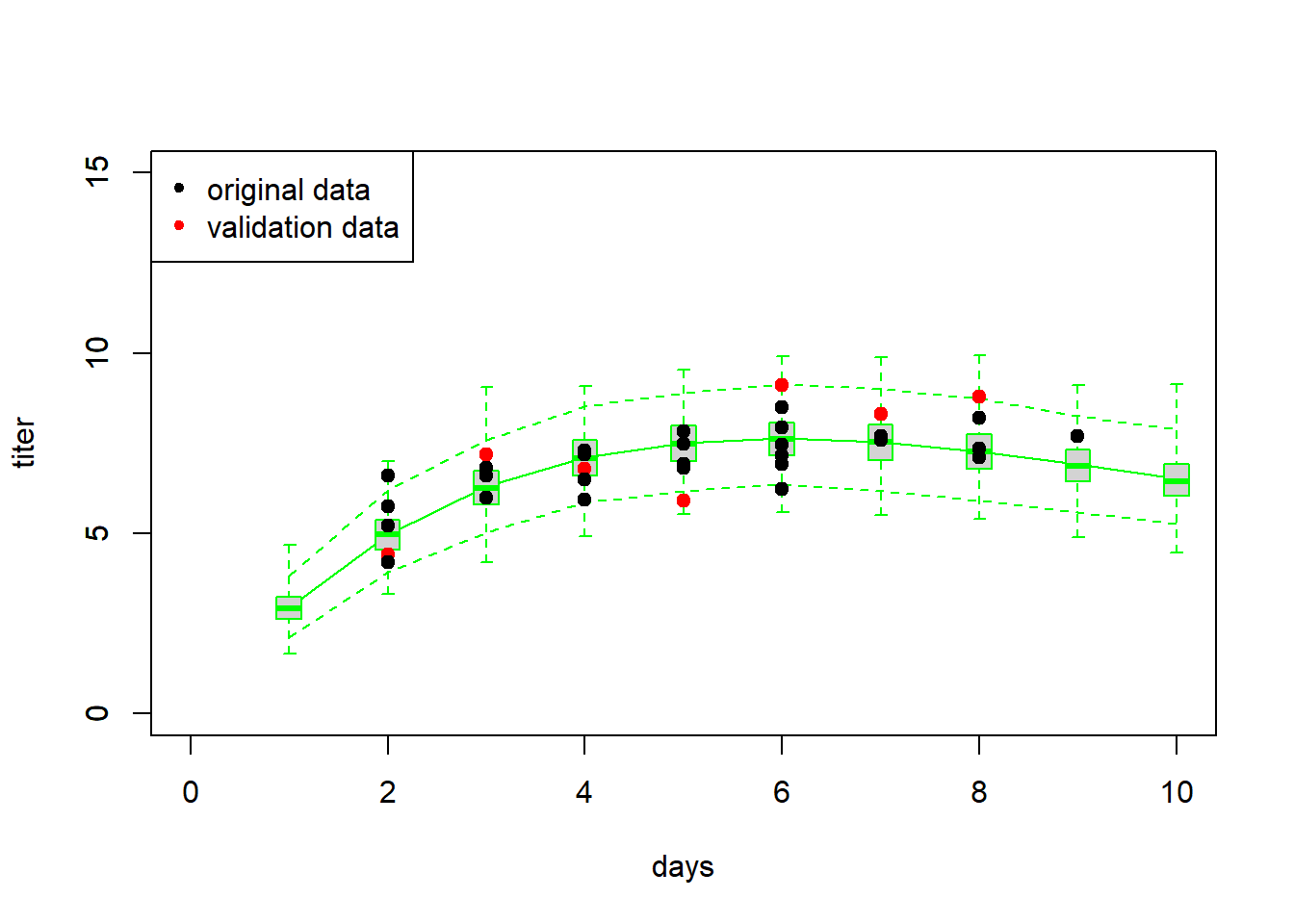Now let’s evaluate the skill of our model at predicting the new data… using our above measures of skill, or performance.

SS_res <- sum((newdata$titer-Ricker(MaxLik$par["a"],MaxLik$par["b"],newdata$day))^2)
SS_tot <- sum((newdata$titer-mean(newdata$titer))^2)
Rsquared_validation <- 1-SS_res/SS_tot

cat("R-squared = ", Rsquared, "\n")
## R-squared =  0.46578
expected <- Ricker(MaxLik$par["a"],MaxLik$par["b"],newdata$day) McFadden_validation <- 1-(sum(dgamma(newdata$titer,shape=MaxLik$par["shape"],scale=expected/MaxLik$par["shape"], log = T))/sum(dgamma(newdata$titer,shape=MaxLik_null$par["shape"],scale=MaxLik_null$par["mean"]/MaxLik_null$par["shape"],log=T)))
cat("pseudo R-squared = ", McFadden_validation, "\n")
## pseudo R-squared =  0.2275702
RMSE = sqrt(mean((newdata$titer-Ricker(MaxLik$par["a"],MaxLik$par["b"],newdata$day))^2))
cat("RMSE = ", RMSE, "\n")
## RMSE =  1.144803

The above analyses seem to indicate that the model fits the new data well, and that the model is successfully able to explain some of the variation in the new data.

### Generality/extrapolation

Imagine we collect some more new data, this time in which titers are measured from day 10 to 16. Let’s see if the model does a good job now!

#########
# Validation #2

newdata <- data.frame(        # imagine these are new observations...
day = c(10,11,12,13,14,15,16),
titer = c(6.8,8.0,4.5,3.1,2.7,1.2,0.04)
)
newdata
##   grade day titer
## 1     1  10  6.80
## 2     1  11  8.00
## 3     1  12  4.50
## 4     1  13  3.10
## 5     1  14  2.70
## 6     1  15  1.20
## 7     1  16  0.04

As before, let’s first simply visualize the new data against the cloud of data possibly produced under the fitted model…

plot(Myx$titer~Myx$day,xlim=c(0,20),ylim=c(0,15),type="n",xlab="days",ylab="titer")
expected <- Ricker(MaxLik$par['a'],MaxLik$par['b'],1:20)
points(1:20,expected,type="l",col="green")

expected <- Ricker(MaxLik$par['a'],MaxLik$par['b'],1:20)
simdata <- array(0,dim=c(1000,20))
for(i in 1:1000){
simdata[i,] <- rgamma(20,shape=MaxLik$par['shape'],scale=expected/MaxLik$par['shape'])
}

upper <- apply(simdata,2,function(t) quantile(t,0.975))
lower <- apply(simdata,2,function(t) quantile(t,0.025))

points(1:20,upper,type="l",col="green",lty=2)
points(1:20,lower,type="l",col="green",lty=2)

points(newdata$day,newdata$titer,cex=1.5,pch=20,col="red")
points(Myx$day,Myx$titer,cex=1.5,pch=20,col="black")
legend("topleft",pch=c(20,20),col=c("black","red"),legend=c("original data","new data"))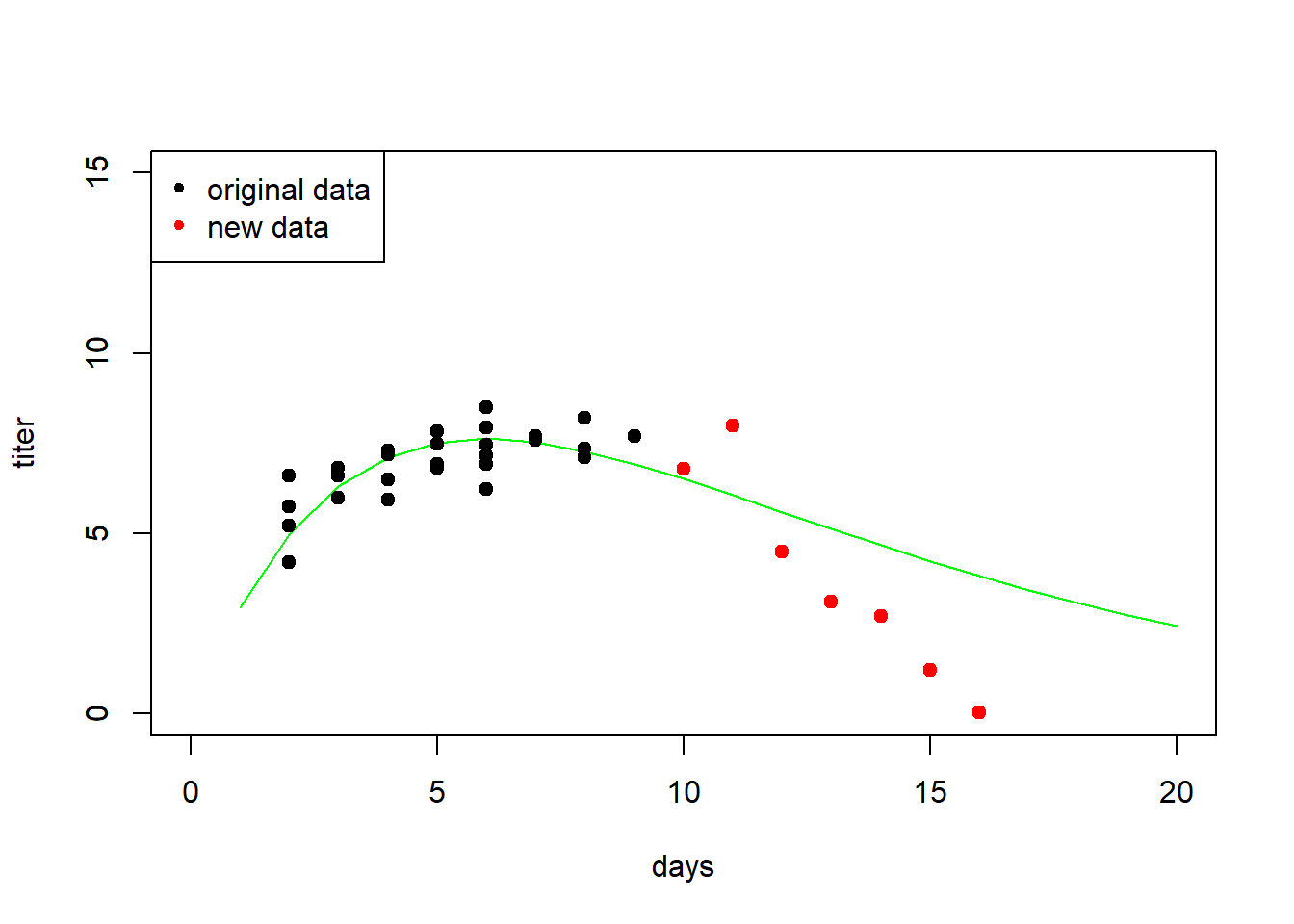Now let’s evaluate the skill of our model at predicting the withheld data… using our favorite measures of skill, or performance.

SS_res <- sum((newdata$titer-Ricker(MaxLik$par["a"],MaxLik$par["b"],newdata$day))^2)
SS_tot <- sum((newdata$titer-mean(newdata$titer))^2)
Rsquared_validation <- 1-SS_res/SS_tot

cat("R-squared = ", Rsquared_validation, "\n")
## R-squared =  0.3208912
expected <- Ricker(MaxLik$par["a"],MaxLik$par["b"],newdata$day) McFadden_validation <- 1-(sum(dgamma(newdata$titer,shape=MaxLik$par["shape"],scale=expected/MaxLik$par["shape"], log = T))/sum(dgamma(newdata$titer,shape=MaxLik_null$par["shape"],scale=MaxLik_null$par["mean"]/MaxLik_null$par["shape"],log=T)))
cat("pseudo R-squared = ", McFadden_validation, "\n")
## pseudo R-squared =  -0.3697861
RMSE = sqrt(mean((newdata$titer-Ricker(MaxLik$par["a"],MaxLik$par["b"],newdata$day))^2))
cat("RMSE = ", RMSE, "\n")
## RMSE =  2.196227

Interestingly, this example shows a couple things:
1) Models are often not very good at extrapolation.
2) The R-squared isn’t always the best measure of model performance.
3) The McFadden pseudo-Rsquared can go below 0!
4) It’s really important to evaluate goodness-of-fit in addition to model performance!

### Cross-validation

In many cases, you will not have new data against which to test the model. Cross-validation allows us to test the model anyway. Here is some pseudocode:

1. Partition the data into k partitions
2. Fit the model, leaving one data partition out at a time
• loop through the partitions.
• for each iteration of the loop, fit the model to all the data EXCEPT the observations in this partition
• use this new fitted model to predict the response variable for all observations in this partition
3. Compute overall model performance for the cross-validation!

The most common forms of cross-validation are: (1) leave-one-out (jackknife) and (10-fold)

Let’s go through an example using the Myxomatosis data!

#####################
# CROSS-VALIDATION
#####################

#####
# PARTITION THE DATA
####

n.folds <- nrow(Myx)   # jackknife

Myx$fold <- sample(c(1:n.folds),size=nrow(Myx),replace=FALSE) init.params <- c(a=1,b=0.2,shape=50) Myx$pred_CV <- 0
for(i in 1:n.folds){
Myx2 <- subset(Myx,fold!=i)   # observations to use for fitting
newfit <- optim(par=init.params, fn=NegLogLik_func, data=Myx2)   # fit the model, leaving out this partition
ndx <- Myx$fold == i Myx$pred_CV[ndx] <- Ricker(newfit$par['a'],newfit$par['b'],Myx$day[ndx]) } Myx$pred_full <- Ricker(MaxLik$par['a'],MaxLik$par['b'],Myx$day) Myx ## grade day titer fold pred_CV pred_full ## 1 1 2 5.207 16 5.037240 5.056415 ## 2 1 2 5.734 23 4.964784 5.056415 ## 3 1 2 6.613 22 4.848091 5.056415 ## 4 1 3 5.997 7 6.421393 6.390345 ## 5 1 3 6.612 14 6.372576 6.390345 ## 6 1 3 6.810 26 6.356336 6.390345 ## 7 1 4 5.930 6 7.239066 7.178825 ## 8 1 4 6.501 24 7.210826 7.178825 ## 9 1 4 7.182 21 7.179047 7.178825 ## 10 1 4 7.292 5 7.173159 7.178825 ## 11 1 5 7.819 12 7.550076 7.560555 ## 12 1 5 7.489 3 7.562292 7.560555 ## 13 1 5 6.918 10 7.585407 7.560555 ## 14 1 5 6.808 19 7.589677 7.560555 ## 15 1 6 6.235 15 7.710027 7.644080 ## 16 1 6 6.916 8 7.678921 7.644080 ## 17 1 2 4.196 1 5.170366 5.056415 ## 18 1 9 7.682 18 6.680056 6.857841 ## 19 1 8 8.189 20 7.113403 7.235101 ## 20 1 7 7.707 13 7.500069 7.513847 ## 21 1 7 7.597 27 7.506437 7.513847 ## 22 1 8 7.112 9 7.251935 7.235101 ## 23 1 8 7.354 25 7.221209 7.235101 ## 24 1 6 7.158 11 7.666217 7.644080 ## 25 1 6 7.466 17 7.651439 7.644080 ## 26 1 6 7.927 4 7.630461 7.644080 ## 27 1 6 8.499 2 7.602640 7.644080 To assess how well the model is performing, let’s compute the root mean squared error for the full model vs the cross-validation: RMSE_full <- sqrt(mean((Myx$titer-Myx$pred_full)^2)) RMSE_CV <- sqrt(mean((Myx$titer-Myx$pred_CV)^2)) RMSE_full ##  0.6767347 RMSE_CV ##  0.7374805 As expected, the RMSE is higher under cross-validation. Is the model still okay? One way to look at this would be to assess the variance explained. VarExplained_full = 1 - mean((Myx$titer-Myx$pred_full)^2)/mean((Myx$titer-mean(Myx$titer))^2) VarExplained_CV = 1 - mean((Myx$titer-Myx$pred_CV)^2)/mean((Myx$titer-mean(Myx$titer))^2) VarExplained_full ##  0.46578 VarExplained_CV ##  0.3655692 Clearly the model performance is lower for the cross-validation, and usually will be! But this is also a more honest evaluation of model performance. ## Example: multiple logistic regression NOTE: example modified from here Let’s evaluate which factors were related to surviving the titanic disaster! ########## # Cross-validation: titanic disaster example! titanic <- read.csv("titanic.csv",header=T) head(titanic) ## PassengerId Survived Pclass ## 1 1 0 3 ## 2 2 1 1 ## 3 3 1 3 ## 4 4 1 1 ## 5 5 0 3 ## 6 6 0 3 ## Name Sex Age SibSp ## 1 Braund, Mr. Owen Harris male 22 1 ## 2 Cumings, Mrs. John Bradley (Florence Briggs Thayer) female 38 1 ## 3 Heikkinen, Miss. Laina female 26 0 ## 4 Futrelle, Mrs. Jacques Heath (Lily May Peel) female 35 1 ## 5 Allen, Mr. William Henry male 35 0 ## 6 Moran, Mr. James male NA 0 ## Parch Ticket Fare Cabin Embarked ## 1 0 A/5 21171 7.2500 S ## 2 0 PC 17599 71.2833 C85 C ## 3 0 STON/O2. 3101282 7.9250 S ## 4 0 113803 53.1000 C123 S ## 5 0 373450 8.0500 S ## 6 0 330877 8.4583 Q Our goal is to model the probability of surviving the titanic disaster as a function of covariates like sex, age, number of siblings or spouses onboard, number of parents or children, passenger fare, etc. Let’s first build a simple logistic regression model for this problem. titanic2 <- na.omit(titanic) model1 <- glm(Survived ~ Sex + scale(Age) + scale(SibSp) + scale(Parch) + scale(Fare), data=titanic2, family="binomial") #logistic regression summary(model1) ## ## Call: ## glm(formula = Survived ~ Sex + scale(Age) + scale(SibSp) + scale(Parch) + ## scale(Fare), family = "binomial", data = titanic2) ## ## Deviance Residuals: ## Min 1Q Median 3Q Max ## -2.5661 -0.6868 -0.5385 0.7323 2.3106 ## ## Coefficients: ## Estimate Std. Error z value Pr(>|z|) ## (Intercept) 1.2071 0.1591 7.587 3.27e-14 *** ## Sexmale -2.5334 0.2052 -12.348 < 2e-16 *** ## scale(Age) -0.3125 0.1043 -2.996 0.002739 ** ## scale(SibSp) -0.3796 0.1140 -3.330 0.000868 *** ## scale(Parch) -0.1984 0.1001 -1.982 0.047523 * ## scale(Fare) 0.9127 0.1663 5.487 4.08e-08 *** ## --- ## Signif. codes: 0 '***' 0.001 '**' 0.01 '*' 0.05 '.' 0.1 ' ' 1 ## ## (Dispersion parameter for binomial family taken to be 1) ## ## Null deviance: 964.52 on 713 degrees of freedom ## Residual deviance: 695.26 on 708 degrees of freedom ## AIC: 707.26 ## ## Number of Fisher Scoring iterations: 5 Alternatively, we could write our own likelihood function!! params <- c( int=1, male = -1, age = 0, sibsp = 0, parch = 0, fare = 0 ) LikFunc <- function(params){ linear <- params['int'] + params['male']*as.numeric(titanic2$Sex=="male") +
params['age']*scale(titanic2$Age) + params['sibsp']*scale(titanic2$SibSp) +
params['parch']*scale(titanic2$Parch) + params['fare']*scale(titanic2$Fare)
logitlinear <-  1/(1+exp(-(linear)))
-sum(dbinom(titanic2$Survived,size=1,prob = logitlinear,log=T)) } LikFunc(params) ##  459.757 MLE <- optim(fn=LikFunc,par = params) MLE$par
##        int       male        age      sibsp      parch       fare
##  1.1988722 -2.5318874 -0.3174421 -0.3816480 -0.2047207  0.9109768

Interesting- the results are very slightly different from the glm results…! (I’m not sure why…)

### Univariate relationships

Let’s visualize the univariate relationships in this model! One way to do this is to hold all other variables at their mean value…

SibSp_range <- range(titanic$SibSp) Parch_range <- range(titanic$Parch)
Fare_range <- range(titanic$Fare) Age_range <- range(titanic$Age,na.rm = T)

First, let’s look at fare

###

plot(titanic$Survived~titanic$Fare,pch=16,xlab="FARE ($)",ylab="Survived!") predict_df <- data.frame( Sex = "male", Age = mean(titanic$Age,na.rm=T),
SibSp = mean(titanic$SibSp), Parch = mean(titanic$Parch),
Fare = seq(Fare_range,Fare_range)
)

probSurv <- predict(model1,predict_df,type="response")

lines(seq(Fare_range,Fare_range),probSurv)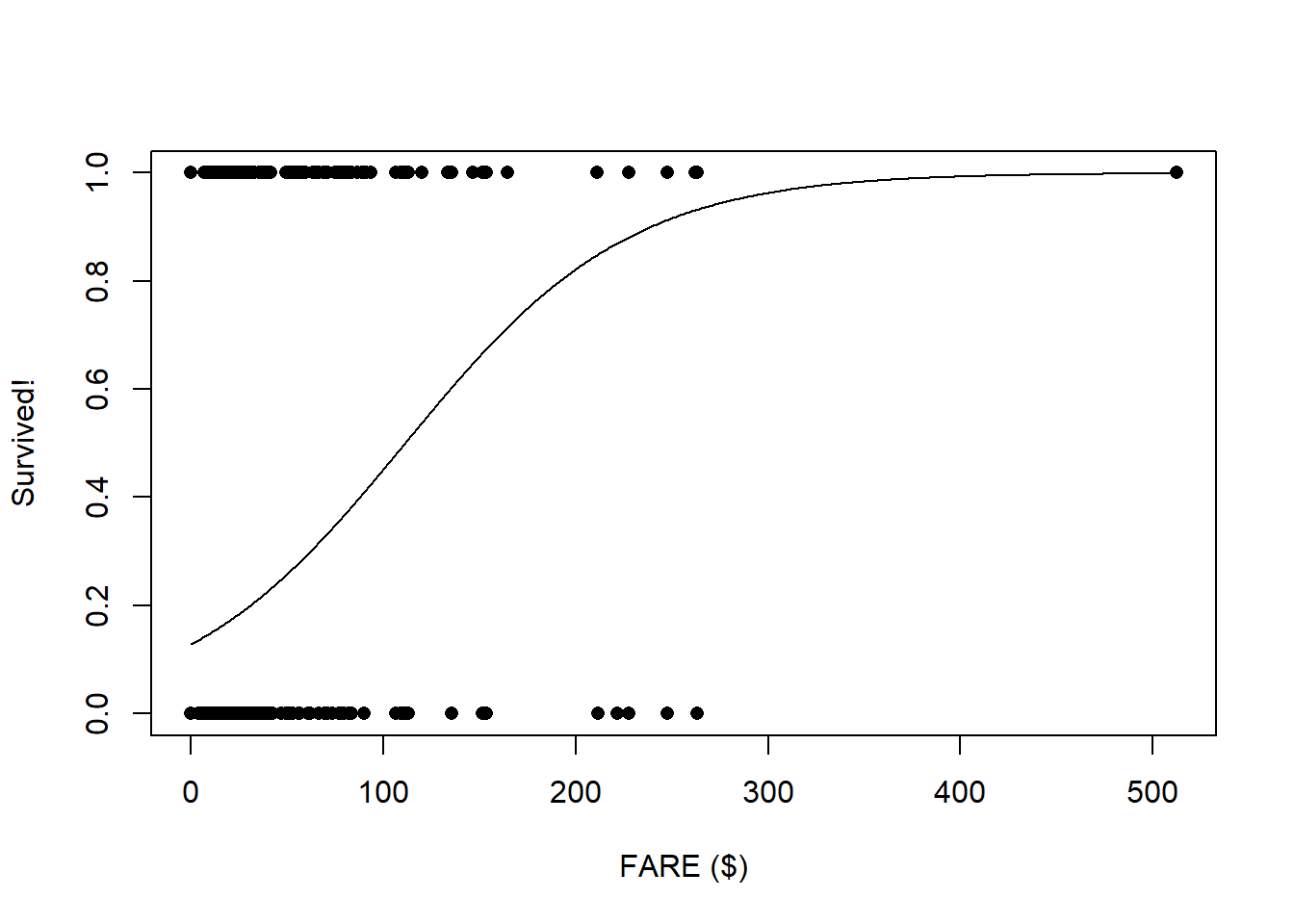Next, let’s look at Sex:

###

predict_df <- data.frame(
Sex = c("male","female"),
Age = mean(titanic$Age,na.rm=T), SibSp = mean(titanic$SibSp),
Parch = mean(titanic$Parch), Fare = mean(titanic$Fare,na.rm=T)
)

tapply(titanic$Survived,titanic$Sex,mean)[2:1]
##      male    female
## 0.1889081 0.7420382
probSurv <- predict(model1,predict_df,type="response")
names(probSurv) <- c("male","female")

probSurv
##      male    female
## 0.2039291 0.7634165

Now, let’s look at age:

###

plot(titanic$Survived~titanic$Age,pch=16,xlab="AGE",ylab="Survived!")

predict_df <- data.frame(
Sex = "male",
Age = seq(Age_range,Age_range),
SibSp = mean(titanic$SibSp), Parch = mean(titanic$Parch),
Fare = mean(titanic$Fare,na.rm=T) ) probSurv <- predict(model1,predict_df,type="response") lines(seq(Age_range,Age_range),probSurv)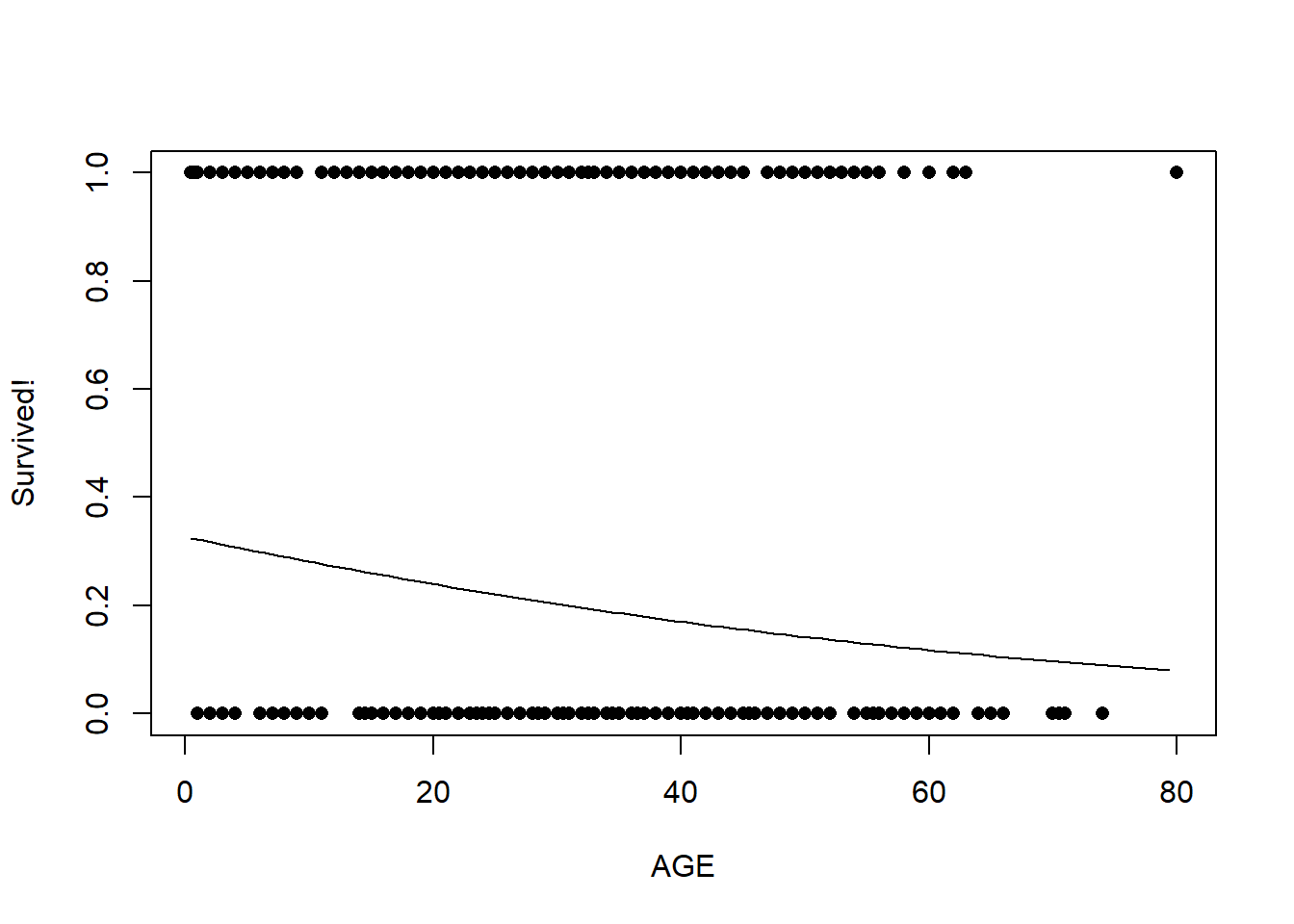Now, let’s look at number of siblings/spouses: ### plot(titanic$Survived~titanic$SibSp,pch=16,xlab="# of Siblings/spouses",ylab="Survived!") predict_df <- data.frame( Sex = "male", Age = mean(titanic$Age,na.rm=T),
SibSp = seq(SibSp_range,SibSp_range,0.01),
Parch = mean(titanic$Parch), Fare = mean(titanic$Fare,na.rm=T)
)

probSurv <- predict(model1,predict_df,type="response")

lines(seq(SibSp_range,SibSp_range,0.01),probSurv)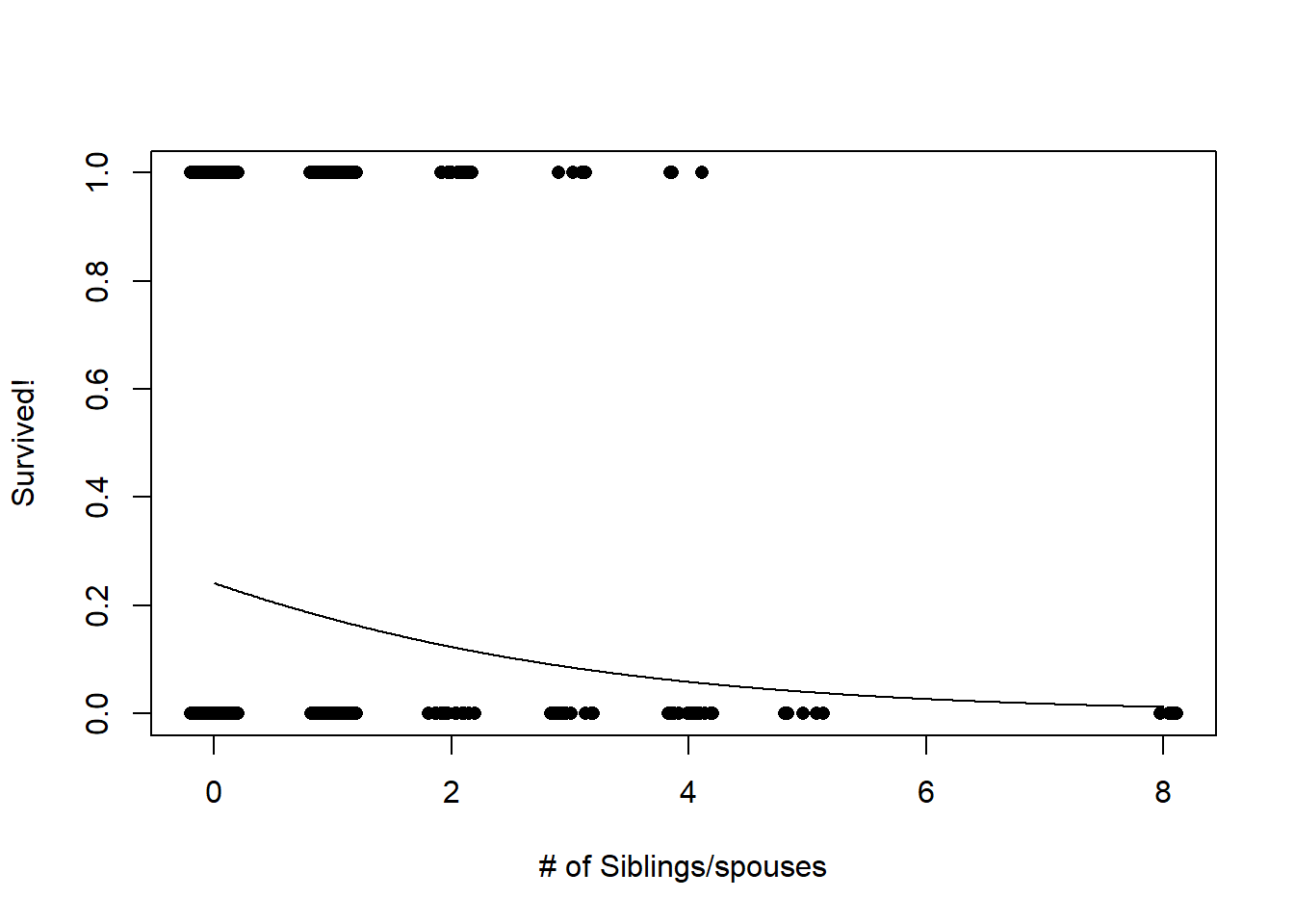### Performance evaluation / validation

Since we are interested in evaluating model performance, let’s see how well the model predicts which people survived the titanic disaster…

First of all, we will use cross-validation to evaluate model predictive performance

BUT… since the response is binary, our model skill metrics don’t really work too well!

For binary responses (0 or 1), the ROC curve (and area under the curve statistic) is a good way of evaluating model performance!

First let’s prepare the workspace- load the packages!

library(ROCR)
## Loading required package: gplots
##
## Attaching package: 'gplots'
## The following object is masked from 'package:stats':
##
##     lowess
library(rms)
## Loading required package: Hmisc
## Loading required package: survival
## Loading required package: Formula
## Loading required package: ggplot2
##
## Attaching package: 'Hmisc'
## The following objects are masked from 'package:base':
##
##     format.pval, units
## Loading required package: SparseM
##
## Attaching package: 'SparseM'
## The following object is masked from 'package:base':
##
##     backsolve
model1 <- glm(Survived ~ Sex + SibSp + Parch + Fare, data=titanic, family="binomial")

Then, we set the number of “folds” for cross-validation

###################################
#################### CROSS VALIDATION CODE FOR BINARY RESPONSE

n.folds = 10       # set the number of "folds"
foldVector = rep(c(1:n.folds),times=floor(length(titanic$Survived)/9))[1:length(titanic$Survived)]

Then, we do the cross validation, looping through each fold of the data, leaving out each fold in turn for model training.

CV_df <- data.frame(
CVprediction = numeric(nrow(titanic)),      # make a data frame for storage
realprediction = 0,
realdata = 0
)

for(i in 1:n.folds){
fit_ndx <- which(foldVector!=i)
validate_ndx <- which(foldVector==i)
model <- glm(formula = Survived ~ Sex + SibSp + Parch + Fare, family = "binomial", data = titanic[fit_ndx,])
CV_df$CVprediction[validate_ndx] <- plogis(predict(model,newdata=titanic[validate_ndx,])) CV_df$realprediction[validate_ndx]  <-  plogis(predict(model1,newdata=titanic[validate_ndx,]))
CV_df$realdata[validate_ndx] <- titanic$Survived[validate_ndx]
}

CV_RMSE = sqrt(mean((CV_df$realdata - CV_df$CVprediction)^2))       # root mean squared error for holdout samples in 10-fold cross-validation
real_RMSE = sqrt(mean((CV_df$realdata - CV_df$realprediction)^2))  # root mean squared error for residuals from final model

# print RMSE statistics

cat("The RMSE for the model under cross-validation is: ", CV_RMSE, "\n")
## The RMSE for the model under cross-validation is:  0.3983029
cat("The RMSE for the model using all data for training is: ", real_RMSE, "\n")
## The RMSE for the model using all data for training is:  0.3937799

However, RMSE doesn’t have much value for binary responses like this! Here we use the ROC curve instead.

### Aside: ROC curve and AUC

ROC is a useful threshold-independent metric for evaluating the performance of a binary classifier!

What does it mean to be threshold independent? It helps to look at the data we have for evaluating model performance

head(CV_df)
##   CVprediction realprediction realdata
## 1    0.1247408      0.1253180        0
## 2    0.8317980      0.8374769        1
## 3    0.7568756      0.7432668        1
## 4    0.7904207      0.7977907        1
## 5    0.1755321      0.1711690        0
## 6    0.1813810      0.1720213        0

To effectively compare our predictions with the observation we have to pick some threshold above which we call the prediction a “1” and below which it is a zero.

A threshold-independent classifier like ROC considers model performance across all possible thresholds.

Here are some example ROC curves: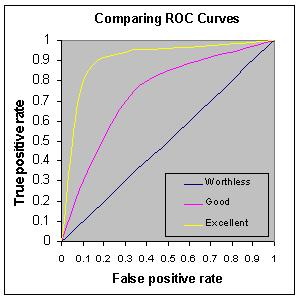The ROC curve is generated by plotting the true positive rate against the false positive rate for all possible thresholds. For example, take the extremes:

Imagine the threshold is zero, so we predict all outcomes are a 1; that is, all people are predicted to survive the titanic disaster. In this case, the true positive rate is exactly 1 (all those that survived were predicted to survive), and the false positive rate (the rate at which mortalities were incorrectly classified as survival) is also 1!

Imagine the opposite case: we predict everyone died. In this case, the true positive rate is 0 (all those that survived were predicted to not survive), as is the false positive rate (all mortalities are correctly classified!)

Now imagine a threshold of around 0.5- we call everything above this a “1”. Now we HOPE that the observations with predictions above 0.5 are enriched in true positives relative to false positives. Maybe we get 80% correct classification of survivors and say 10 incorrect classification of mortalities (false positives).

Q What would a perfect classifier look like?

Q What would a classifier look like that performed no better than random chance?

Q What if the classifier performed worse than random chance?

Now, let’s consider the meaning of the area under the ROC curve. This is an important skill metric for a binary classifier!

Okay, back to the example! Let’s plot out the ROC curves!

par(mfrow=c(2,1))
pred <- prediction(CV_df$CVprediction,CV_df$realdata)     # for holdout samples in cross-validation
perf <- performance(pred,"tpr","fpr")
auc <- performance(pred,"auc")
plot(perf, main="Cross-validation")
text(.9,.1,paste("AUC = ",round(auc@y.values[],2),sep=""))

pred <- prediction(CV_df$realprediction,CV_df$realdata)     # for final model
perf <- performance(pred,"tpr","fpr")
auc <- performance(pred,"auc")
plot(perf, main="All data")
text(.9,.1,paste("AUC = ",round(auc@y.values[],2),sep=""))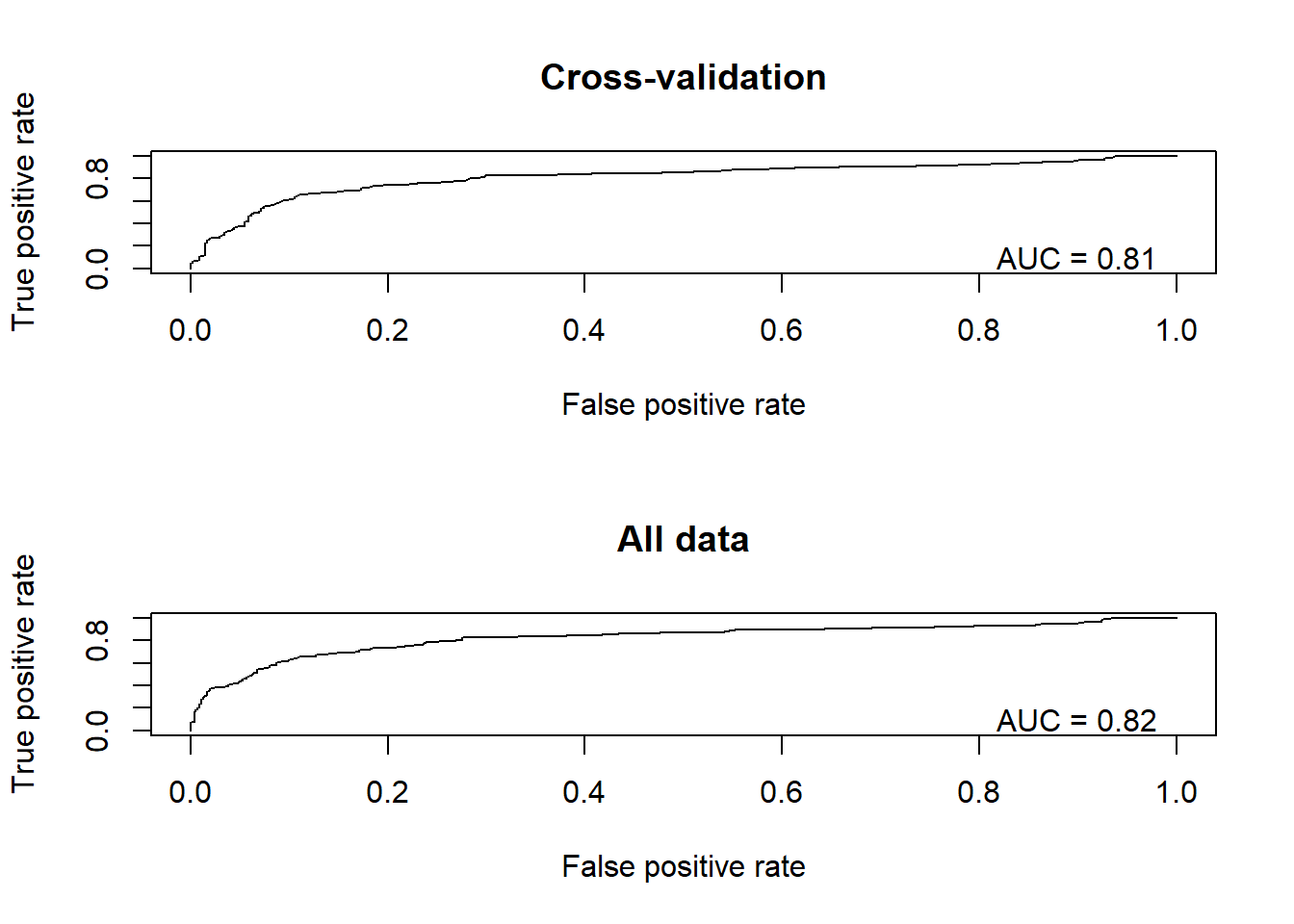Finally, we can use the same pseudo-R-squared metric we learned above as an alternative metric of performance

CV_df$CVprediction[which(CV_df$CVprediction==1)] <- 0.9999       # ensure that all predictions are not exactly 0 or 1
CV_df$CVprediction[which(CV_df$CVprediction==0)] <- 0.0001
CV_df$realprediction[which(CV_df$realprediction==1)] <- 0.9999
CV_df$realprediction[which(CV_df$realprediction==0)] <- 0.0001

fit_deviance_CV <- mean(-2*(dbinom(CV_df$realdata,1,CV_df$CVprediction,log=T)-dbinom(CV_df$realdata,1,CV_df$realdata,log=T)))
fit_deviance_real <- mean(-2*(dbinom(CV_df$realdata,1,CV_df$realprediction,log=T)-dbinom(CV_df$realdata,1,CV_df$realdata,log=T)))
null_deviance <- mean(-2*(dbinom(CV_df$realdata,1,mean(CV_df$realdata),log=T)-dbinom(CV_df$realdata,1,CV_df$realdata,log=T)))
deviance_explained_CV <- (null_deviance-fit_deviance_CV)/null_deviance   # based on holdout samples
deviance_explained_real <- (null_deviance-fit_deviance_real)/null_deviance   # based on full model...

# print RMSE statistics

cat("The McFadden R2 for the model under cross-validation is: ", deviance_explained_CV, "\n")
## The McFadden R2 for the model under cross-validation is:  0.2564415
cat("The McFadden R2 for the model using all data for training is: ", deviance_explained_real, "\n")
## The McFadden R2 for the model using all data for training is:  0.2760832

–go to next lecture–